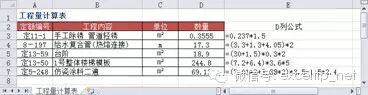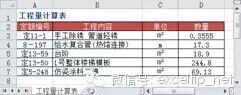# 如何在公式中添加注解

﻿﻿﻿﻿﻿﻿19万粉丝共同关注→ 解决方案：

→ 操作方法

=0.237*1.5+N("管道周长×管道长")

=(3.3+1.3+4.05)*2+N("管道长×管道数量")

=((30+1.5)&T(N("台阶周长")))*(0.3&T(N("台阶高")))*(2&T(N("台阶个数")))

=((7.2+6.4)&T(N("楼梯水平长")))*(3.6&T(N("楼梯宽度")))*(5&T(N("层数")))

=((7.01*2+3.38*2)&T(N("房间周长")))*(3.5&T(N("房间净高度")))-(1.5*2.4&T(N("门窗洞口面积")))→ 原理分析

1．加0法：+N("注解文字")，利用N函数可以将文本值转换为0的特性，将“注解文字”转换为0，即相当于在原公式中+0，达到注解公式的目的，如D3、D4单元格公式所示。

2．合并空文本法：&T(N("注解文字"))，使用T、N函数组合，将“注解文字”转换为空文本，即相当于在原公式中的各项目之后合并一个空文本，达到注解公式的目的，如D5、D6、D7单元格公式所示，此方法得到的结果为文本值。

N函数用于过滤文本，T函数用于过滤数值。语法如下：

N(value)

T(value)

 数值或引用 N函数返回值 T函数返回值 数值 原数值 空文本"" 日期 该日期的序列号 空文本"" TRUE 1 空文本"" FALSE 0 空文本"" 错误值，例如 #DIV/0! 错误值 错误值 文本 0 原文本

Office交流 QQ 群